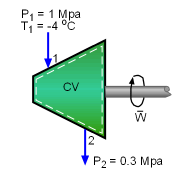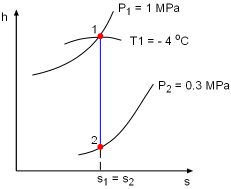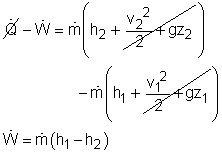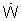Ch 6. Entropy Multimedia Engineering Thermodynamics Entropy Tds Relations EntropyChange IsentropicProcess IsentropicEfficiency EntropyBalance (1) EntropyBalance (2) ReversibleWork
 Chapter 1. Basics 2. Pure Substances 3. First Law 4. Energy Analysis 5. Second Law 6. Entropy 7. Exergy Analysis 8. Gas Power Cyc 9. Brayton Cycle 10. Rankine Cycle Appendix Basic Math Units Thermo Tables Search eBooks Dynamics Fluids Math Mechanics Statics Thermodynamics Author(s): Meirong Huang Kurt Gramoll ©Kurt GramollTHERMODYNAMICS - CASE STUDY SOLUTION

A turbine replaces a throttling valve to reduce the pressure of liquid refrigerant-134a from 1 MPa to 0.3 MPa. The power generated by the turbine and the money saved from this energy conversion need to be determined.

Assumptions:

• The pressure reduction process is reversible.
• Kinetic and potential energies are negligible.Schematic of the TurbinePressure Reducing process on the
h-s Diagram

 Saturated R-134a Temperature Table Saturated R-134a Pressure Table Superheated Steam Table

(1) Determine the power generated by the turbine

Take the turbine as a control volume. The inlet is state 1 and the exit is state 2.

Since the assumptions state that the turbine is adiabatic and the process is reversible, this means the process is an isentropic process (will be introduced in the following section). Hence,

S1 = S2

The turbine has only one inlet and one exit. That gives

s1 = s2

where
s = entropy per unit mass

The saturation temperature of the refrigerant R-134a at 1 MPa is 39.39 oC. Hence, the refrigerant R-134a at 1 MPa and -4oC is a compressed liquid. In the absence of compressed liquid data, the properties of compressed liquid can be approximated by the saturated liquid data. At -4oC, the saturated properties of refrigerant R-134 are

Psat = 0.25274 MPa
vf = 0.0007644 m3/kg
hf = 44.75 kJ/kg
s = 0.1777 kJ/(kg-K)

The properties of state 1 are:

h1 = 44.75 + 0.0007644( 1- 0.25274)(103)
= 45.32 kJ/kg
s1 = 0.1777 kJ/(kg-K)
v1 = 0.0007644 m3/kg

At state 2, the pressure and the entropy are known, hence, enthalpy of state 2 can be determined as

h2 = 44.75 + 0.0007644( 0.3- 0.25274)(103)
= 44.75 kJ/kg

The energy balance of the turbine is:substituting the mass flow rate and the enthalpies into the above equation yields= 200(45.32-44.75) = 114 kW

(2) Determine how much the turbine can save the facility per year

If the turbine operate 8,760 hour per year, the amount of power produced per year is

Wyr = 114( 8760) = 998,640 kWh/yr

If the price of the electricity is \$0.08/kWh, then 998,640 kWh of electricity is worth of

998,640 (0.08) = \$79,891

The facility can save \$79,891 if the throttling valve is replaced by a turbine.